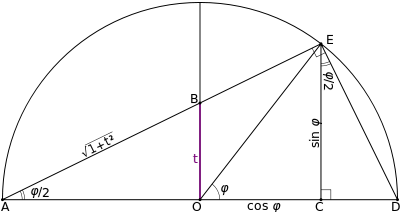# Tangent half-angle formula

In trigonometry, tangent half-angle formulas relate the tangent of half of an angle to trigonometric functions of the entire angle. Among these are the followingFrom these one can derive identities expressing the sine, cosine, and tangent as functions of tangents of half-angles:## Proofs

### Algebraic proofs

Use double-angle formulae and sin2 α + cos2 α = 1,thenQ.E.D.

Also, using the angle addition and subtraction formulae for both the sine and cosine one obtains:Pairwise addition of the above four formulae yields:Settingandand substituting yields:Dividing the sum of sines by the sum of cosines one arrives at:### Geometric proofsA geometric proof of the tangent half-angle formula

Applying the formulae derived above to the rhombus figure on the right, it is readily shown thatIn the unit circle, application of the above shows that. According to similar triangles,. It follows thatThe sides of this rhombus have length 1. The angle between the horizontal line and the shown diagonal is (a + b)/2. This is a geometric way to prove a tangent half-angle formula. Note that sin((a + b)/2) and cos((a + b)/2) just show their relation to the diagonal, not the real value.

## The tangent half-angle substitution in integral calculus

In various applications of trigonometry, it is useful to rewrite the trigonometric functions (such as sine and cosine) in terms of rational functions of a new variable t. These identities are known collectively as the tangent half-angle formulae because of the definition of t. These identities can be useful in calculus for converting rational functions in sine and cosine to functions of t in order to find their antiderivatives.

Technically, the existence of the tangent half-angle formulae stems from the fact that the circle is an algebraic curve of genus 0. One then expects that the circular functions should be reducible to rational functions.

Geometrically, the construction goes like this: for any point (cos φ, sin φ) on the unit circle, draw the line passing through it and the point (−1, 0). This point crosses the y-axis at some point y = t. One can show using simple geometry that t = tan(φ/2). The equation for the drawn line is y = (1 + x)t. The equation for the intersection of the line and circle is then a quadratic equation involving t. The two solutions to this equation are (−1, 0) and (cos φ, sin φ). This allows us to write the latter as rational functions of t (solutions are given below).

Note also that the parameter t represents the stereographic projection of the point (cos φ, sin φ) onto the y-axis with the center of projection at (−1, 0). Thus, the tangent half-angle formulae give conversions between the stereographic coordinate t on the unit circle and the standard angular coordinate φ.

Then we haveandBy eliminating phi between the directly above and the initial definition of t, one arrives at the following useful relationship for the arctangent in terms of the natural logarithmIn calculus, the Weierstrass substitution is used to find antiderivatives of rational functions of sin φ and cos φ. After settingThis implies thatfor some integer n, and therefore## Hyperbolic identities

One can play an entirely analogous game with the hyperbolic functions. A point on (the right branch of) a hyperbola is given by (cosh θ, sinh θ). Projecting this onto y-axis from the center (−1, 0) gives the following:with the identitiesandThe use of this substitution for finding antiderivatives was introduced by Karl Weierstrass.

Finding θ in terms of t leads to following relationship between the hyperbolic arctangent and the natural logarithm:("ar-" is used rather than "arc-" because "arc" is about arc length and "ar" abbreviates "area". It is the area between two rays and a hyperbola, rather than the arc length between two rays measure along an arc a circle.)

## The Gudermannian function

Main article: Gudermannian function

Comparing the hyperbolic identities to the circular ones, one notices that they involve the same functions of t, just permuted. If we identify the parameter t in both cases we arrive at a relationship between the circular functions and the hyperbolic ones. That is, ifthenwhere gd(θ) is the Gudermannian function. The Gudermannian function gives a direct relationship between the circular functions and the hyperbolic ones that does not involve complex numbers. The above descriptions of the tangent half-angle formulae (projection the unit circle and standard hyperbola onto the y-axis) give a geometric interpretation of this function.

## Pythagorean triples

Main article: Pythagorean triple

The tangent of half of an acute angle of a right triangle whose sides are a Pythagorean triple will necessarily be a rational number in the interval (0, 1). Vice versa, when a half-angle tangent is a rational number in the interval (0, 1), there is a right triangle that has the full angle and that has side lengths that are a Pythagorean triple.